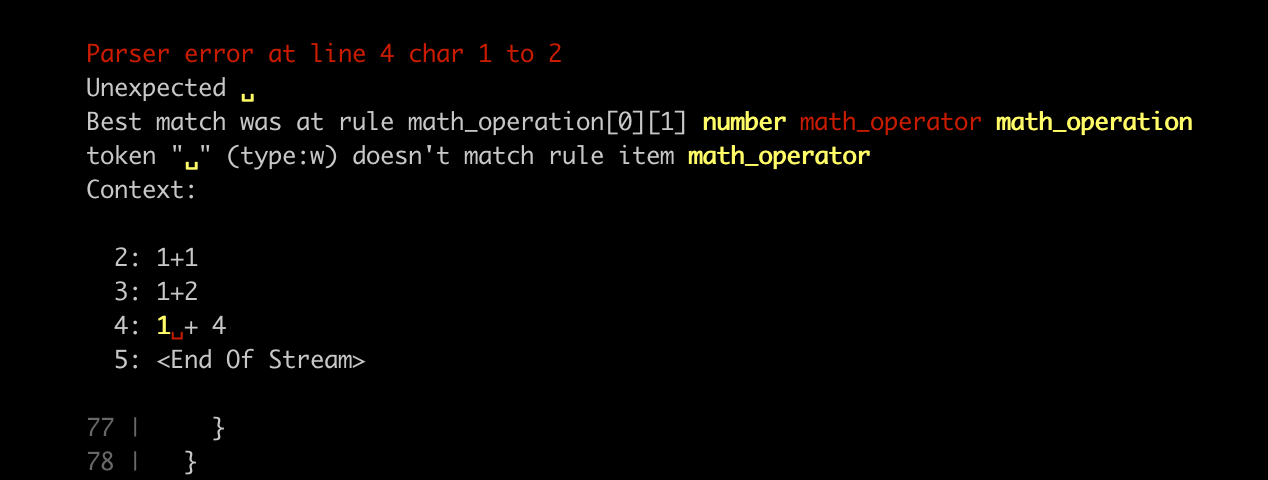Nerdiest Political Manifesto

# npm

## meta-parser-generator

1.0.4 • Public • Published

# Meta Parser Generator

`npm install meta-parser-generator`

Meta Parser Generator will help you generate an efficient parser using a grammar and a token definition. Meta programming is used to generate a single self contained parser file.

This code has been extracted from https://github.com/batiste/blop-language

## Characteristics

• LL parser (Left to Right parser), arbitrary look ahead
• Direct Left recursion support (no indirect)
• Parser code is generated from a grammar
• Good parsing performance (provided your grammar is efficient)
• Decent error reporting on parsing error
• Memoization
• Small source code (~500 lines of code), no dependencies

## How to generate and use a parser

This will generate a mathematical operation parser

```const { generateParser } = require('meta-parser-generator');
const path = require('path');

// only 3 possible tokens
const tokensDefinition = {
'number': { reg: /^[0-9]+(\.[0-9]*)?/ },
'math_operator': { reg: /^(\+|-|\*|%)/ },
'newline': { str: '\n' }
}

const grammar = {
// START is the convention keyword for the entry point of the grammar
'START': [
// necessary to accept the first line be MATH
['MATH', 'LINE*', 'EOS'], // EOS is the End Of Stream token, added automatically by the tokenizer
// * is the repeating modifier {0,∞}. Better than recursion as it does not use the call stack
['LINE*', 'EOS'],
],
'LINE': [
// we define a line as always starting with a newline
['newline', 'MATH'],
['newline'],
],
'MATH': [
// direct left recursion here
['MATH', 'math_operator', 'number'],
['number'],
],
};

// this generate the executable parser file
generateParser(grammar, tokensDefinition, path.resolve(__dirname, './parser.js'));
console.log('parser generated');```

Then you can use the generated parser this way

```const parser = require('./parser');
const { tokensDefinition, grammar } = require('./generator');
const { displayError } = require('meta-parser-generator');

function parse(input) {
const tokens = parser.tokenize(tokensDefinition, input);
const ast = parser.parse(tokens);
if (!ast.success) {
displayError(tokens, tokensDefinition, grammar, ast);
}
return ast;
}

let ast = parse('9+10-190.3');
console.log(ast)```

### How does generated parser works?

Each grammar rule you write is transformed into a function, and those grammar functions call each other until the input parsing is sucessful. Therefor the JavaScript call stack is used by the generated parser. So if you design a very recursive grammar, you might trigger a "Maximum call stack size exceeded" error for a large input.

In our example `MATH` grammar rule above you have a left recursion. It means you can parse expressions such as 1+2+3+4+5+...X, where X is the maximum stack size of V8.

To know the default maximum stack size of V8 you can run `node --v8-options | grep stack-size`. If the default size is not enough for your grammar, use this option to extend the size. You can also try to rewrite your grammar in order to be less recursive.

Anything that can be handled by a modifier rather than recursion will not use the call stack and should be preffered.

### AST interface

```type ASTNode = RuleNode | Token

interface RuleNode {
stream_index: number                // position of the first token for this rule in the token stream
type: str                           // name of the rule
subRule: number                     // index of this grammar rule in the subrule array
children: [ASTNode]                 // list of children
named: { [key: string]: ASTNode; }  // named elements withing this rule, see named aliases
}```

At the leaf of the AST you will find the final tokens. They have a slightly different interface

```interface Token {
stream_index: number // position of the token in the token stream
type: str            // name of token
value: str           // the value of the token
len: number          // shortcut for value.length
lineStart: number    // line start position in the input
columnStart: number  // column start position in the input
start: number        // character start position in the input
}```

### Modifiers

There is 3 modifiers you can add at the end of a rule or token

``````* is the {0,∞} repeating modifier
+ is the {1,∞} repeating modifier
? is the {0,1} conditional modifier
``````

#### Example

`['PREPOSITION', 'ADJECTIVE*', 'NAME']`

### Named alias

To facilitate your work with the AST, you can name a rule or a token using a colon

```'MATH': [
['MATH', 'math_operator:operator', 'number:num'], // math_operator and number token are named
['number:num'],                                   // here only number is named
]```

Then in the corresponding `RuleNode` you will find the `math_operator` in the children, but also in the named object. This is useful to more easily handle and differenciate your grammar rules:

```// a function that handle both MATH grammar rules defined above
function handle_MATH_node(node: RuleNode) {
const named = node.named
// if there is an operator, we are dealing with sub rule 0
if(named['operator']) {
const left_recursion = handle_MATH_node(node.children)
console.log(`\${left_recursion} \${named['operator'].value} \${named['num'].value}`)
} else {
console.log(named['num'].value)
}
}```

### Errors

The util function `displayError` will display detailed informations about a tokenizer or parsing error. The hint given is based on the first grammar rule found that consume the most token from the stream.## Keywords

### Install

`npm i meta-parser-generator`

### Repository

github.com/batiste/meta-parser-generator

5

1.0.4